Monday, February 17, 2014

ggplot2: Cheatsheet for Visualizing Distributions

>> Histograms

In the third and last of the ggplot series, this post will go over interesting ways to visualize the distribution of your data. I will make up some data, and make sure to set the seed.

library(ggplot2)
library(gridExtra)
set.seed(10005)

xvar <- c(rnorm(1500, mean = -1), rnorm(1500, mean = 1.5))
yvar <- c(rnorm(1500, mean = 1), rnorm(1500, mean = 1.5))
zvar <- as.factor(c(rep(1, 1500), rep(2, 1500)))
xy <- data.frame(xvar, yvar, zvar)

>> Histograms

I've already done a post on histograms using base R, so I won't spend too much time on them. Here are the basics of doing them in ggplot. More on all options for histograms here.

The R cookbook has a nice page about it too: http://www.cookbook-r.com/Graphs/Plotting_distributions_(ggplot2)/

Also, I found this really great aggregation of all of the possible geom layers and options you can add to a plot. In general the site is a great reference for all things ggplot.

#counts on y-axis
g1<-ggplot(xy, aes(xvar)) + geom_histogram()                                      #horribly ugly default
g2<-ggplot(xy, aes(xvar)) + geom_histogram(binwidth=1)                            #change binwidth
g3<-ggplot(xy, aes(xvar)) + geom_histogram(fill=NA, color="black") + theme_bw()   #nicer looking

#density on y-axis
g4<-ggplot(xy, aes(x=xvar)) + geom_histogram(aes(y = ..density..), color="black", fill=NA) + theme_bw()

grid.arrange(g1, g2, g3, g4, nrow=1)
## stat_bin: binwidth defaulted to range/30. Use 'binwidth = x' to adjust
## this. stat_bin: binwidth defaulted to range/30. Use 'binwidth = x' to
## adjust this. stat_bin: binwidth defaulted to range/30. Use 'binwidth = x'
## to adjust this.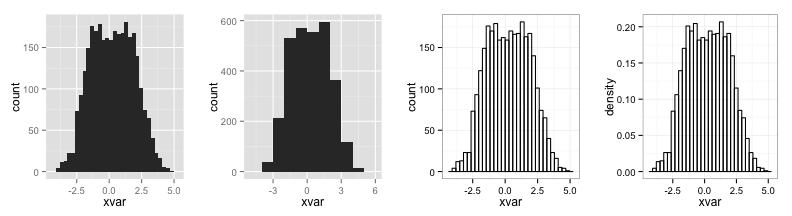Notice the warnings about the default binwidth that always is reported unless you specify it yourself. I will remove the warnings from all plots that follow to conserve space.

>> Density plots

We can do basic density plots as well. Note that the default for the smoothing kernel is gaussian, and you can change it to a number of different options, including kernel=“epanechnikov” and kernel=“rectangular” or whatever you want. You can find all of those options here.

#basic density
p1<-ggplot(xy, aes(xvar)) + geom_density()

#histogram with density line overlaid
p2<-ggplot(xy, aes(x=xvar)) +
geom_histogram(aes(y = ..density..), color="black", fill=NA) +
geom_density(color="blue")

#split and color by third variable, alpha fades the color a bit
p3<-ggplot(xy, aes(xvar, fill = zvar)) + geom_density(alpha = 0.2)

grid.arrange(p1, p2, p3, nrow=1)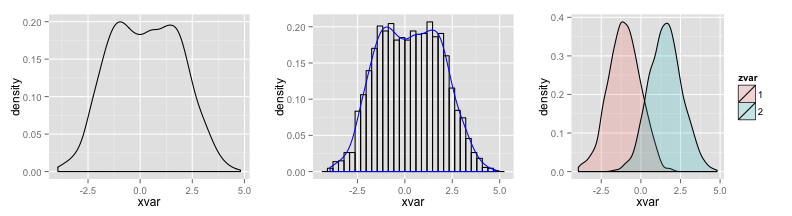>> Boxplots and more

We can also look at other ways to visualize our distributions. Boxplots are probably the most useful in order to describe the statistics of a distribution, but sometimes other visualizations are nice. I show a jitter plot and a violin plot. More on boxplots here. Note that I removed the legend from each one because it is redundant.

#boxplot
b1<-ggplot(xy, aes(zvar, xvar)) +
geom_boxplot(aes(fill = zvar)) +
theme(legend.position = "none")

#jitter plot
b2<-ggplot(xy, aes(zvar, xvar)) +
geom_jitter(alpha=I(1/4), aes(color=zvar)) +
theme(legend.position = "none")

#violin plot
b3<-ggplot(xy, aes(x = xvar)) +
stat_density(aes(ymax = ..density..,  ymin = -..density..,
fill = zvar, color = zvar),
geom = "ribbon", position = "identity") +
facet_grid(. ~ zvar) +
coord_flip() +
theme(legend.position = "none")

grid.arrange(b1, b2, b3, nrow=1)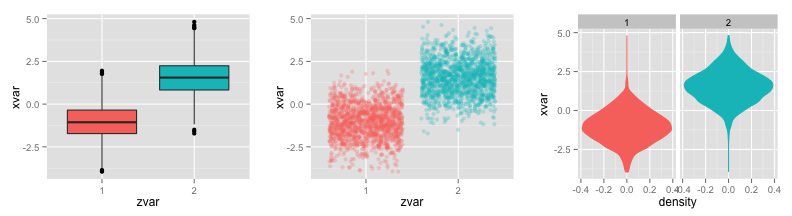>> Putting multiple plots together

Finally, it's nice to put different plots together to get a real sense of the data. We can make a scatterplot of the data, and add marginal density plots to each side. Most of the code below I adapted from this StackOverflow page.

One way to do this is to add distribution information to a scatterplot as a “rug plot”. It adds a little tick mark for every point in your data projected onto the axis.

#rug plot
ggplot(xy,aes(xvar,yvar))  + geom_point() + geom_rug(col="darkred",alpha=.1)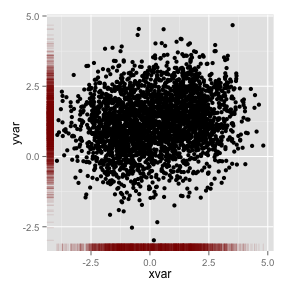Another way to do this is to add histograms or density plots or boxplots to the sides of a scatterplot. I followed the stackoverflow page, but let me know if you have suggestions on a better way to do this, especially without the use of the empty plot as a place-holder.

I do the density plots by the zvar variable to highlight the differences in the two groups.

#placeholder plot - prints nothing at all
empty <- ggplot()+geom_point(aes(1,1), colour="white") +
theme(
plot.background = element_blank(),
panel.grid.major = element_blank(),
panel.grid.minor = element_blank(),
panel.border = element_blank(),
panel.background = element_blank(),
axis.title.x = element_blank(),
axis.title.y = element_blank(),
axis.text.x = element_blank(),
axis.text.y = element_blank(),
axis.ticks = element_blank()
)

#scatterplot of x and y variables
scatter <- ggplot(xy,aes(xvar, yvar)) +
geom_point(aes(color=zvar)) +
scale_color_manual(values = c("orange", "purple")) +
theme(legend.position=c(1,1),legend.justification=c(1,1))

#marginal density of x - plot on top
plot_top <- ggplot(xy, aes(xvar, fill=zvar)) +
geom_density(alpha=.5) +
scale_fill_manual(values = c("orange", "purple")) +
theme(legend.position = "none")

#marginal density of y - plot on the right
plot_right <- ggplot(xy, aes(yvar, fill=zvar)) +
geom_density(alpha=.5) +
coord_flip() +
scale_fill_manual(values = c("orange", "purple")) +
theme(legend.position = "none")

#arrange the plots together, with appropriate height and width for each row and column
grid.arrange(plot_top, empty, scatter, plot_right, ncol=2, nrow=2, widths=c(4, 1), heights=c(1, 4))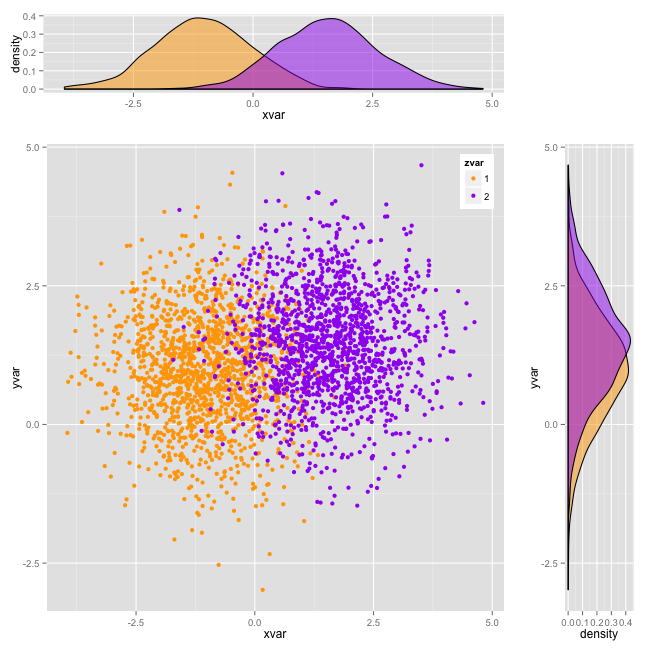It's really nice that grid.arrange() clips the plots together so that the scales are automatically the same. You could get rid of the redundant axis labels by adding in theme(axis.title.x = element_blank()) in the density plot code. I think it comes out looking very nice, with not a ton of effort. You could also add linear regression lines and confidence intervals to the scatterplot. Check out my first ggplot2 cheatsheet for scatterplots if you need a refresher.

1.Wow, Slawa this is a great resource! Thanks so much for putting this together.

Also I think 3rd plot under 'Boxplots' is a not a volcano plot, but "violin plot":
http://en.wikipedia.org/wiki/Violin_plot

Great stuff.

1.Thanks! Thanks for the comment; I changed it.

2.You should check out beanplots, which are basically violin plots, with superimposed boxplots and dot plots. There is a beanplot package for R, but ggplot2 does not include a geom specifically for this. You can easily create one by using geom_violin, geom_boxplot, and geom_point.

1.Yes, I think that's really the beauty of ggplot2 and what I've tried to convey over three posts about it is the idea of layering. You can superimpose layers of points, boxplots, and whatever else you want very easily once you know how to build the different components.

3.> "It's really nice that grid.arrange() clips the plots together so that the scales are automatically the same. "

That's not the case, and for this very reason I wouldn't recommend using grid.arrange when the axes ought to be aligned. Consider using gtable instead, e.g http://stackoverflow.com/a/21531303/471093

4.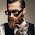I like this blog
Thank you for sharing.
hichem

5.Such a great post, Slawa!
Tips for the readers - if you are interested in customizing your graphs in ggplot, checkout this blog post in R bloggers - http://www.r-bloggers.com/how-to-customize-ggplot2-graphics/

6.That is an extremely smart written article. I will be sure to bookmark it and return to learn extra of your useful information. Thank you for the post. I will certainly return.

Personalized Kids Scrubs
Toddler scrubs

7.add insight into a broad and obtain useful information thanks admin.http://jualjaketkulit.co.id visit back my website

Note: Only a member of this blog may post a comment.